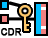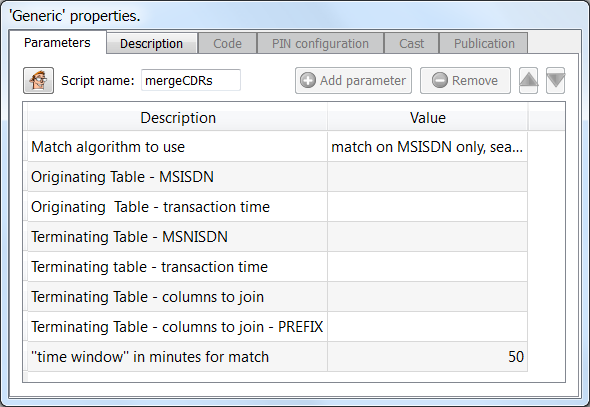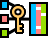﻿ 5. Detailed description of the Actions > 5.4. Join Tables > 5.4.6. Merge CDRs

# 5.4.6. Merge CDRs

Icon:Function: mergeCDRs

Property window:Short description:

Perform a “fuzzy” join between the 2 input tables.

Long Description:

A typical Mobile Money transaction goes, basically, the following way:

oa subscriber A wants to transfer some money to another subscriber B.

othe subscriber A sends a SMS to the central SMS server.

This SMS is crypted and contains:

a)the amount to transfer.

b)the MSISDN from B.

othe whole transaction is logged inside 2 different tables:

a)Each row of the “TABLE A” describes one money transfer between 2 subscribers identified by their MSISDN number. The columns are MSISDN_A, MSISDN_B, TRANSACTION_TIME, TRANSACTION_AMOUNT.

b)Each row of the “TABLE B” describes one SMS between a subscriber MSISDN_A and the central SMS server. The columns are MSISDN, TIME, ANTENNA_ID.

We want to find, for each transaction of TABLE A, the ANTENNA_ID from the subscriber that started the transaction. In other word, we need to compute a join between the TABLE A and the TABLE B.

We could try to compute the join using the standardJoin Action. More precisely:

2.We use the columns (MSISDN_A, TRANSACTION_TIME) from “TABLE A” as Composite-Primary Key.

3.We use the columns (MSISDN, TIME) from “TABLE B” as Composite-Primary Key.

This approach is described in more detail in section 5.1.6 about Composite primary Keys. This naïve approach will fail because the “TIME” column is not exactly the same inside each of the two tables (because these are two different servers that are generating the two tables A and B and their clock is not tightly synchronized).

To be able to compute the join, we need a “Fuzzy” match on the TIME dimension. Meaning that, if the TRANSATION_TIME and the TIME are separated by less than 3 minutes (this is the “Time Window” parameter), we’ll decide that the TIME columns are “matching” (and we’ll thus produce a join and retrieve the ANTENNA_ID associated with the transaction).

The parameters of theMergeCDR Action are:

4.The algorithm used to compute the Fuzzy match.

5.The Originating Table: the Table A: the Transaction Table.

6.The Terminating Table: the Table B: the SMS Table.

7.The Time window (in the example above: 3 minutes).

TheMergeCDR Action is written in JavaScript so you can easily tweak/modify it to suit your specific needs.